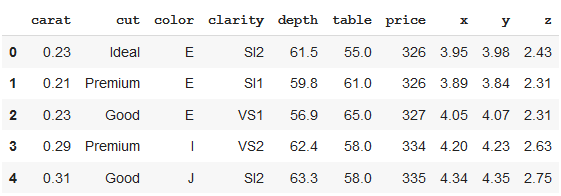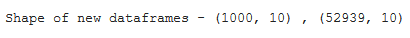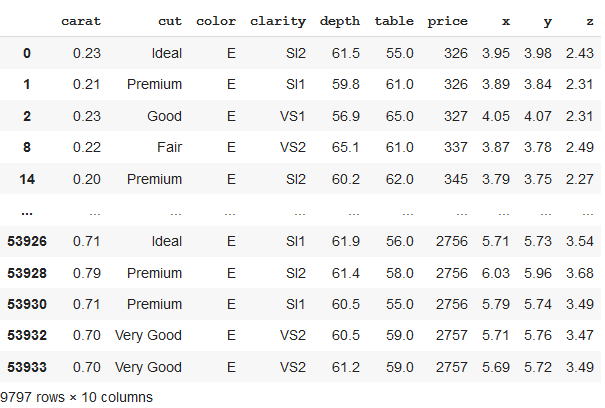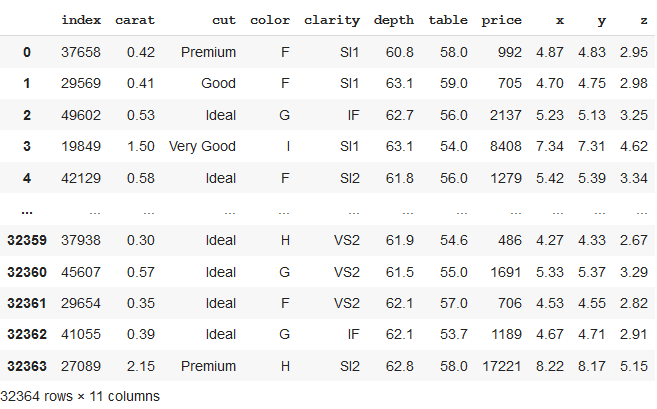# Split Pandas Dataframe by Rows

• Difficulty Level : Medium
• Last Updated : 29 Aug, 2020

We can try different approaches for splitting Dataframe to get the desired results. Let’s take an example of a dataset of diamonds.

Attention geek! Strengthen your foundations with the Python Programming Foundation Course and learn the basics.

To begin with, your interview preparations Enhance your Data Structures concepts with the Python DS Course. And to begin with your Machine Learning Journey, join the Machine Learning - Basic Level Course

 `# importing libraries``import` `seaborn as sns``import` `pandas as pd``import` `numpy as np`` ` `# data needs not to be downloaded separately``df  ``=` `sns.load_dataset(``'diamonds'``)``df.head()`

Output:Method 1: Splitting Pandas Dataframe by row index

In the below code, the dataframe is divided into two parts, first 1000 rows, and remaining rows. We can see the shape of the newly formed dataframes as the output of the given code.

 `# splitting dataframe by row index``df_1 ``=` `df.iloc[:``1000``,:]``df_2 ``=` `df.iloc[``1001``:,:]``print``(``"Shape of new dataframes - {} , {}"``.``format``(df_1.shape, df_2.shape))`

Output:Method 2: Splitting Pandas Dataframe by groups formed from unique column values

Here, we will first grouped the data by column value “color”. The newly formed dataframe consists of grouped data with color = “E”.

 `# splitting dataframe by groups``# grouping by particular dataframe column``grouped ``=` `df.groupby(df.color)``df_new ``=` `grouped.get_group(``"E"``)``df_new`

Output:Method 3 : Splitting Pandas Dataframe in predetermined sized chunks

In the above code, we can see that we have formed a new dataset of a size of 0.6 i.e. 60% of total rows (or length of the dataset), which now consists of 32364 rows. These rows are selected randomly.

 `# splitting dataframe in a particular size``df_split ``=` `df.sample(frac``=``0.6``,random_state``=``200``)``df_split.reset_index()`

Output:My Personal Notes arrow_drop_up